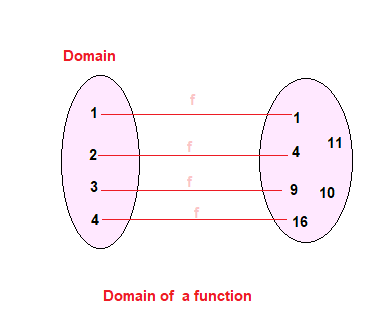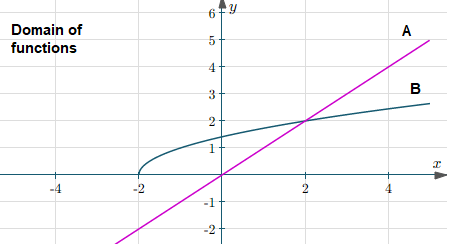# Domain of a Function

## Domain of a Function

For a function $f: A \rightarrow B$
Set A is called the domain of the function f
Set B is the called the codomain of the functionFor real function, A and B are subset of the real numbers.
In some cases,domain of the real function may not be explicity defined. We are just given the function
y=f(x)
In such cases domain would mean the set of real values of x for which f(x) assumes real values.Domain of the function f is denoted by D(f)
Therefore,
D(f) = {$x \in R$, f(x) is a real number}

Example
1. $y=x^2$
Solution
Here it is clear that y assumes real values for all $x \in R$
So, D(y) =R

2. Let A= {2,4,5,6}. A function f is defined from X to N
$f={(x,y),y=x^3 + 2, x \in A ,y \in N }$
Find the domain and codomain of the function?
Solution
Domain will be Set A
D(f) ={2,4,5,6}
Codomain will be N

## How to find the domain of a function algebraically

This can be divided into two section

## How to find the domain of a function algebraically

• Find the values of x for which given real function takes the below forms
a. $\frac {1}{0}$
This is the case used when to find the domain of a function with a fraction
b. $\sqrt {a \; negative \; integer}$
This is the case used when to find the domain of a function with a squareroot
c. $\frac {1}{\sqrt {a \; negative \; integer}}$
d. $\frac {0}{0}$
e. $log (a \; negative \; integer)$
f. $\frac {1} {log (a \; negative \; integer) \; or (\; equal \; to \; 1)}$
• The set of real numbers excluding those obtained in step 1 is the domain of a function

### How to find the domain of a function using graph

We can find the domain using graph also. Here domain is the x-values taken by the graphHere Domain of Function of the graph A is $(-\infty,\infty)$ Here Domain of function of the graph B is $[-2,\infty)$

## How do you write the domain in interval notation

 $(a,b)$ It is the open interval set between point and b such that All the points between a and b belong to the open interval (a, b) but a, b themselves do not belong to this interval $\{ y:a < y < b\}$ $[a,b]$ It is the closed interval set between point and b such that All the points between a and b belong to the open interval (a, b) including a, b $\{ x:a \le x \le b\}$ $[a,b)$ It is the open interval set between point and b such that All the points between a and b belong to the open interval (a, b) including a, but not b $\{ x:a \le x < b\}$ $(a,b)$ It is the open interval set between point and b such that All the points between a and b belong to the open interval (a, b) including b, but not a $\{ x:a < x \le b\}$

## Solved Examples on How to find the domain of a function

Find the domain of the below functions
1. $y =f(x) = \frac {1}{x-1}$
Solution

Here we see that function is defined for all values of $x \in R$ except for x=1 (it becomes of the form 1/0 for x=1) .
So domain will be given
D(f) = R -1

2. $f(x) = \frac {1}{ \sqrt {x -3}}$
Solution
The function is defined only
x -3 > 0 or x > 3
Domain will be given as
D(f) =$(3,\infty )$

3. $f(x) = \frac {1}{x^2 + 3}$
Solution
Since $x^2 + 3$ > 0 for $x \in R$, This function is defined for all values of $x \in R$
D(f) =$(- \infty,\infty )$

4.$f(x) = \sqrt {(x-3)(x-4)}$
Solution
Here for f(x) to be real,
$(x-3)(x-4) \geq 0$
or $x \in (-\infty ,3] \cup [4,\infty]$
So domain of the function will be
D(f) = $(-\infty ,3] \cup [4,\infty]$

### Quiz Time on domain of a function

Question 1.
which is of the below relation is not a function.
A. R = {(2,2),(2,4),(3,3), (4,4)}
B. R = {(2,1),(3,1), (4,2)}
C. R = {(1,2),(2,3),(3,4), (4,5), (5,6), (6,7)}
D. None of the above
Question 2.
Let A = {1, 2, 3,4} and B = {5, 7}. Then possible number of relation from A to B ?
A. 64
B. 256
C. 16
D. 32
Question 3.
if function f : X -> R, $f (x) = x^3$, where X = {-1, 0, 3, 9, 7}, the function expressed in ordered pair will be
A. {(-1,-1),(1,1),(3,27), (7,343),(9,729)}
B. {(-1,-1),(0,0),(3,27)}
C. {(-1,1),(0,0),(3,9), (7,343),(9,729)}
D. {(-1,-1),(0,0),(3,27), (7,343),(9,729)}
Question 4.
Let f(x) =x2 ,find the value of $\frac {f(2.1) -f(2)}{2.1 -2}$
A. 4
B. .41
C. 4.1
D. .14
Question 5.
Find the domain of the function defined as $f(x)=\sqrt {9-x^2}$
A. [-3,3]
B. [0,3]
C.(0,3)
D.[-3,0)
Question 6.
Find the domain of the function $f(x) =\frac {x^2+1}{x^2-5x+4}$
A. R
B. R - {4}
C. R - {1}
D. R -{1,4}